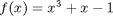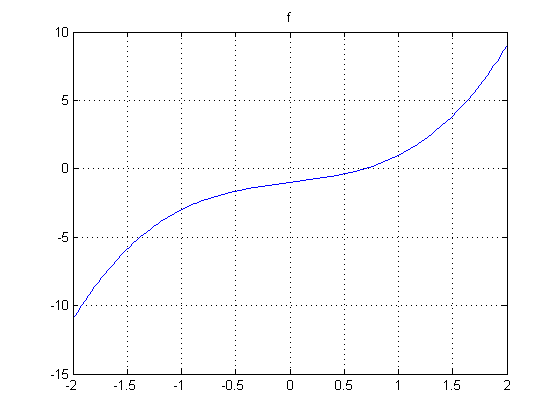# Rooting Around in MATLAB – Part 1

I've been interested in teaching for a long time, including ways to use MATLAB. One concept that students might need to understand early in their college careers is that of finding roots or zeros of functions. To understand at least some of the algorithms, you might want to teach the students about fixed points for functions. It's the basis for some methods of solving equations or finding roots, algorithms such as Newton's method, finding square roots, and more.

### Example FunctionHere's one common way to represent this polynomial in MATLAB, using coefficients of descending powers of the independent variable.

p = [1 0 1 -1];

I can then use polyval to evaluate the polynomial. And then I can plot it.

x = -2:0.1:2;
y = polyval(p,x);
plot(x,y)
title f
gridI could also represent the polynomial as an anonymous function and plot it with fplot.

f = @(x) x.^3 + x - 1;
fplot(f,[-2 2])
title f
grid on### Find the Roots or Zeros

I have at least 2 choices in MATLAB for finding a zero or root of this polynomial. The first is to use roots to get all the possible zeros.

rsolution = roots([1 0 1 -1])
rsolution =
-0.34116 +     1.1615i
-0.34116 -     1.1615i
0.68233


You can see that this polynomial has one real root between 0 and 1, and 2 complex roots.

You can also use fzero, one of MATLAB's optimization functions, to find the value. Here we'll select 0.5 as the initial guess.

fzsolution = fzero(f,0.5)
fzsolution =
0.68233


In the next post, I'll describe a way to solve this same problem using an algorithm based on fixed point iteration.

### The Series of Posts

In addition to this post, there will be two more. Until they are published, the following two links will not be available.

Published with MATLAB® 7.8

|